Courses

# NCERT Solutions(Part-3)- Cubes and Cube Roots Class 8 Notes | EduRev

## Class 8 Mathematics by Full Circle

Created by: Full Circle

## Class 8 : NCERT Solutions(Part-3)- Cubes and Cube Roots Class 8 Notes | EduRev

The document NCERT Solutions(Part-3)- Cubes and Cube Roots Class 8 Notes | EduRev is a part of the Class 8 Course Class 8 Mathematics by Full Circle.
All you need of Class 8 at this link: Class 8

Exercise 7.2

Question 1. Find the cube root of each of the following numbers by prime factorisation method.

(i) 64 (ii) 512 (iii) 10648 (iv) 27000 (v) 15625
(vi) 13824 (vii) 110592 (viii) 46656 (ix) 175616 (x) 91125

Solution:

(i) By prime factorisation, we have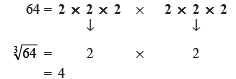Thus, cube root of 64 is 4.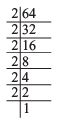(ii) By prime factorisation, we have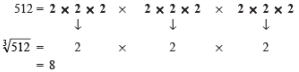Thus,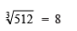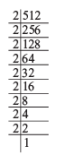(iii) By prime factorisation, we have

∴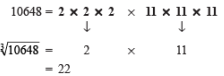Thus, the cube root of 10648 is 22.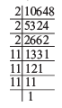(iv) By prime factorisation, we have

∴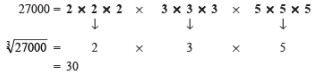Thus, the cube root of 27000 is 30.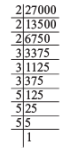(v) By prime factorisation, we have

∴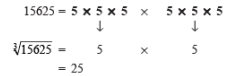Thus, cube root of 15625 is 25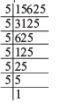(vi) By prime factorisation, we have

13824 = 2 * 2 * 2 * 2 * 2 * 2 * 2 * 2 * 2 * 3 * 3 * 3

∴ ∛13824 = 2 * 2 * 2 * 3

= 24

Thus, the cube root of 13824 is 24.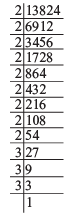(vii) By prime factorisation, we have

110592 = 2 * 2 * 2 * 2 * 2 * 2 * 2 * 2 * 2 * 2 * 2 * 2 * 3 * 3 * 3

∴ ∛110592 = 2 * 2 * 2 * 2 * 3 = 48

Thus, the cube root of 110592 is 48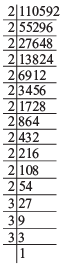(viii) By the prime factorisation, we have

46656 = 2 * 2 * 2 * 2 * 2 * 2 * 3 * 3 * 3 * 3 * 3 * 3

∴ ∛46656 = 2 * 2 * 3 * 3 = 36

Thus, the cube root of 46656 is 32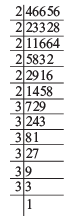(ix) By prime factorisation, we have

175616 = 2 * 2 * 2 * 2 * 2 * 2 * 2 * 2 * 2 * 7 * 7 * 7

= 2 * 2 * 2 * 7 = 56

Thus the cube root of 175616 is 56.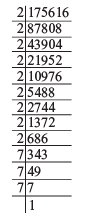(x) By prime factorisation, we have:

91125 = 3 * 3 * 3 * 3 * 3 * 3 * 5 * 5 * 5

∴  ∛91125 = 3 * 3 * 5 = 45

Thus, the cube root of 91125 is 45.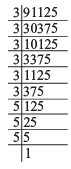Question 2. State true or false.

(i) Cube of any odd number is even.

(ii) A perfect cube does not end with two zeros.

(iii) If square of a number ends with 5, then its cube ends with 25.

(iv) There is no perfect cube which ends with 8.

(v) The cube of a two digit number may be a three digit number.

(vi) The cube of a two digit number may have seven or more digits.

(vii) The cube of a single digit number may be a single digit number.

Solution: (i) False (ii) True (iii) False (iv ) False
(v) False (vi) False (vii) True

Question 3. You are told that 1,331 is a perfect cube. Can you guess without factorisation what is its cube root? Similarly, guess the cube roots of 4913, 12167, 32768.

Solution:

(i) Separating the given number (1331) into two groups:

1331 → 1 and 331

∵ 331 end in 1.

∴ Unit’s digit of the cube root = 1

∵ 13 = 1 and ∛1 = 1

∴ Ten’s digit of the cube root = 1

∴ ∛1331 = 11

(ii) Separating the given number (4913) in two groups:

4913 → 4 and 913

Unit’s digits:

∵ Unit’s digit in 913 is 3.

∴ Unit’s digit of the cube root = 7

[73 = 343; which ends in 3]

Ten’s digit:

∵ 13 = 1, 23 = 8

and 1 < 4 < 8

i.e. 1< 4 < 23

∴ The ten’s digit of the cube root is 1.

∴ ∛4913 = 17

(iii) Separating 12167 in two groups:

12167 → 12 and 167

Unit’s digit:

∵ 167 is ending in 7 and cube of a number ending in 3 ends in 7.

∴ The unit’s digit of the cube root = 3

Ten’s digit:

∵ 23 = 8 and 33 = 27

Also, 8 < 12 < 27

or 23 < 12 < 32

∴ The tens digit of the cube root can be 2.

Thus,  ∛12167 = 23.

(iv) Separating 32768 in two groups:

32768 → 32 and 786

Unit’s digit:

768 will guess the unit’s digit in the cube root.

∵ 768 ends in 8.

∴ Unit’s digit in the cube root = 2

Ten’s digit:

∵ 33 = 27 and 43 – 64

Also, 27 < 32 < 64

or 33 < 32 < 43

∴ The ten’s digit of the cube root = 3.

Thus, ∛32768 = 32

93 docs|16 tests

,

,

,

,

,

,

,

,

,

,

,

,

,

,

,

,

,

,

,

,

,

;